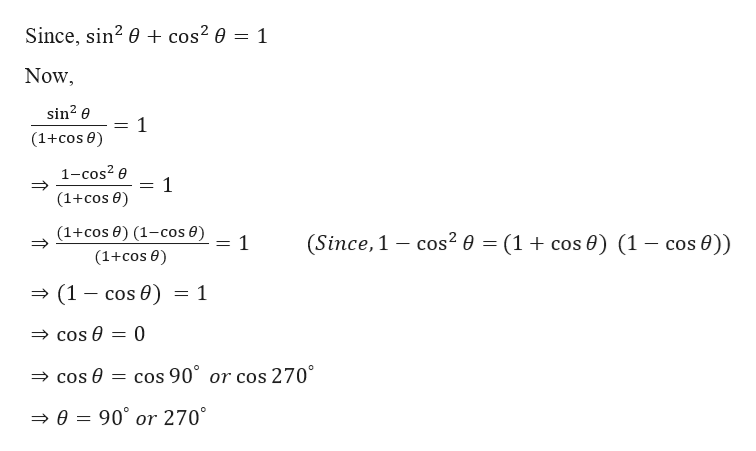# Solve algebraically for all values of θ in the interval  0≤θ<360 that satisfy the equation sin^2θ/(1+cosθ)=1

Question
1 views

Solve algebraically for all values of θ in the interval  0≤θ<360 that satisfy the equation sin^2θ/(1+cosθ)=1

check_circle

Step 1

Given,

Step 2

Now,

...help_outlineImage TranscriptioncloseSince, sin? 0 + cos² 0 = 1 Now, sin? e (1+cos 0) 1-cos? e (1+cos 0) (1+cos 0) (1-cos €) (1+cos 0) (Since, 1 – cos² 0 = (1+ cos 0) (1 – cos 0)) = 1 → (1 – cos 0) = cos 0 = 0 = cos 90° or cos 270° » cos 0 → 0 = 90° or 270° fullscreen

### Want to see the full answer?

See Solution

#### Want to see this answer and more?

Solutions are written by subject experts who are available 24/7. Questions are typically answered within 1 hour.*

See Solution
*Response times may vary by subject and question.
Tagged in
MathAlgebra

### Other